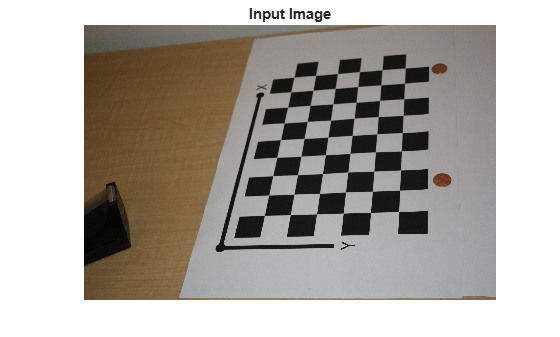# cameraMatrix

Camera projection matrix

## Syntax

``camMatrix = cameraMatrix(cameraParams,rotationMatrix,translationVector)``

## Description

example

````camMatrix = cameraMatrix(cameraParams,rotationMatrix,translationVector)` returns a 4-by-3 camera projection matrix. You can use this matrix to project 3-D world points in homogeneous coordinates into an image.```

## Examples

collapse all

Create a set of calibration images.

```images = imageDatastore(fullfile(toolboxdir('vision'),'visiondata', ... 'calibration','slr'));```

Detect the checkerboard corners in the images.

`[imagePoints,boardSize] = detectCheckerboardPoints(images.Files);`

Generate the world coordinates of the checkerboard corners in the pattern-centric coordinate system, with the upper-left corner at (0,0). The square size is in millimeters.

```squareSize = 29; worldPoints = generateCheckerboardPoints(boardSize,squareSize);```

Calibrate the camera.

```I = readimage(images,1); imageSize = [size(I,1),size(I,2)]; cameraParams = estimateCameraParameters(imagePoints,worldPoints, ... 'ImageSize',imageSize);```

```imOrig = imread(fullfile(matlabroot,'toolbox','vision','visiondata', ... 'calibration','slr','image9.jpg')); figure; imshow(imOrig); title('Input Image');```Undistort image.

`im = undistortImage(imOrig,cameraParams);`

Find reference object in new image.

`[imagePoints,boardSize] = detectCheckerboardPoints(im);`

Compute new extrinsics.

```[rotationMatrix,translationVector] = extrinsics(... imagePoints,worldPoints,cameraParams);```

Calculate camera matrix

`P = cameraMatrix(cameraParams,rotationMatrix,translationVector)`
```P = 4×3 105 × 0.0157 -0.0271 0.0000 0.0404 -0.0046 -0.0000 0.0199 0.0387 0.0000 8.9399 9.4399 0.0072 ```

## Input Arguments

collapse all

Camera parameters, specified as a `cameraParameters` or `cameraIntrinsics` object. You can return the `cameraParameters` object using the `estimateCameraParameters` function. The `cameraParameters` object contains the intrinsic, extrinsic, and lens distortion parameters of a camera.

Rotation of camera, specified as a 3-by-3 matrix. You can obtain this matrix using the `extrinsics` function. You can also obtain the matrix using the `relativeCameraPose` function by transposing its `orientation` output. The `rotationMatrix` and `translationVector` inputs must be real, nonsparse, and of the same class.

Translation of camera, specified as a 1-by-3 vector. The translation vector describes the transformation from the world coordinates to the camera coordinates. You can obtain this vector using the `extrinsics` function. You can also obtain the vector using the `location` and `orientation` outputs of the `relativeCameraPose` function:

• `translationVector` = -`relativeLocation` * `relativeOrientation'`

The `translationVector` inputs must be real, nonsparse, and of the same class.

## Output Arguments

collapse all

Camera projection matrix, returned as a 4-by-3 matrix. The matrix contains the 3-D world points in homogenous coordinates that are projected into the image. When you set `rotationMatrix` and `translationVector` to `double`, the function returns `camMatrix` as `double`. Otherwise it returns `camMatrix` as `single`.

The function computes `camMatrix` as follows:

`camMatrix` = [`rotationMatrix`; `translationVector`] × K.
K: the intrinsic matrix

Then, using the camera matrix and homogeneous coordinates, you can project a world point onto the image.

w × [x,y,1] = [X,Y,Z,1] × `camMatrix`.

 (X,Y,Z): world coordinates of a point (x,y): coordinates of the corresponding image point w: arbitrary scale factor

Data Types: `single` | `double`

## Extended Capabilities

Introduced in R2014b